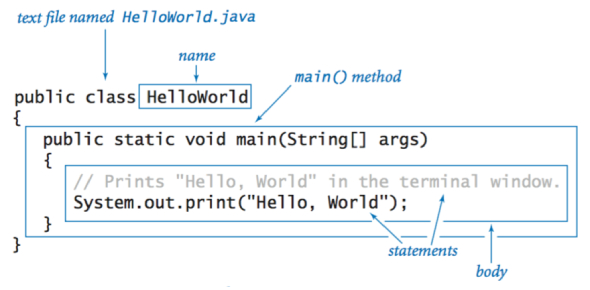# Java Basic Programming Trivia: Quiz!

10 Questions | Total Attempts: 22Settings.

• 1.
Consider the following code: public class TestOne { public static void main(String args[]) { byte x = 3; byte y = 5; System.out.print((y%x) + ", "); System.out.println(y == ((y/x) *x +(y%x))); } } Which of the following gives the valid output for above?
• A.

Prints 2, false

• B.

Prints: 1, true

• C.

Prints: 2, true

• D.

Prints: 1, true

• 2.
Consider the following program: class A extends Thread { public A(Runnable r) {} public void run() { System.out.print("A"); } } class B implements Runnable { public void run(){ System.out.print("B"); } } class C{ public static void main(String[] args) { new A(new B()).start(); } } What will be the output of the above program?
• A.

Compiletime Error

• B.

Prints: AB

• C.

Prints: AB

• D.

Prints: A

• 3.
Dd3)6) Consider the following code: public class Code17 { public static void main(String args[]) { new Code17(); } { System.out.print("Planet "); } { System.out.print("Welcome "); } } Which of the following will be the valid output for the above code?
• A.

Compilation Error

• B.

Planet

• C.

Planet Welcome

• D.

Welcome Planet

• 4.
Polymorphism enables you to:
• A.

Program in the general

• B.

Program in the specific.

• C.

Absorb attributes and behavior from previous classes.

• D.

Hide information from the user.

• 5.
Polymorphism allows for specifics to be dealt with during:
• A.

Execution.

• B.

Compilation.

• C.

Programming.

• D.

Debugging.

• 6.
A(n) class cannot be instantiated.
• A.

Final.

• B.

Concrete.

• C.

Abstract.

• D.

Polymorphic.

• 7.
It is a UML convention to denote the name of an abstract class in:
• A.

Bold.

• B.

italics.

• C.

a diamond.

• D.

There is no convention of the UML to denote abstract classes—they are listed just as any other class.

• 8.
When a subclass constructor calls its superclass constructor, what happens if the superclass’s constructor does not assign a value to an instance variable?
• A.

A syntax error occurs.

• B.

A compile-time error occurs

• C.

A run-time error occurs.

• D.

The program compiles and runs because the instance variables are initialized to their default values.

• 9.
Consider the abstract superclass below: public abstract class Foo { private int a; public int b; public Foo( int aVal, int bVal ) { a = aVal; b = bVal; } // end Foo constructor public abstract int calculate(); } // end class Foo Any concrete subclass that extends class Foo:
• A.

Must implement a method called calculate.

• B.

Will not be able to access the instance variable a.

• C.

Neither (a) nor (b).

• D.

Both (a) and (b)

• 10.
Every object in Java knows its own class and can access this information through method
• A.

GetClass.

• B.

GetInformation.

• C.

ObjectClass.

• D.

ObjectInformation

Related TopicsBack to top